﻿Variance

# Variance

The Variance (Variance) (var) is a measurement of the spread between numbers in a data set relative to its mean. Variance is always non-negative: a small variance indicates that the data points tend to be very close to the mean value and hence to each other, while a high variance indicates that the data points are very spread out around the mean and from each other.Calculation

In the case of a set of N values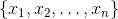and the arithmetic mean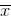, the sample (unbiased) variance is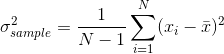The population variance is differ only in division factor: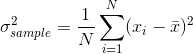Chart Example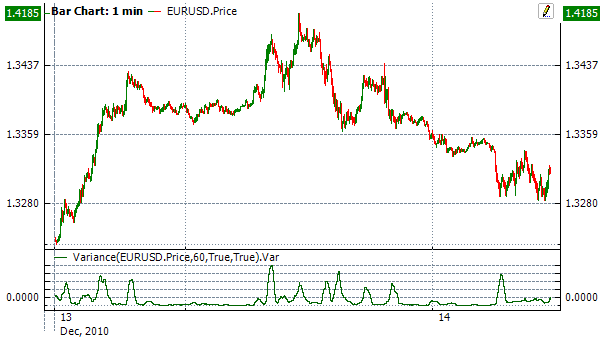Implementation and Usage

To initialize indicator, use one of the following constructors:

Variance – set default values: period = 14

Variance(Int32, Boolean) – set value for period

Variance(TimeSpan, Boolean) – sets time period

Use

Var - property to get current valueExample
C#
``` 1// Create new instance
2var indicator = new Variance(28);
3
4// Number of stored values
5indicator.HistoryCapacity = 2;
6